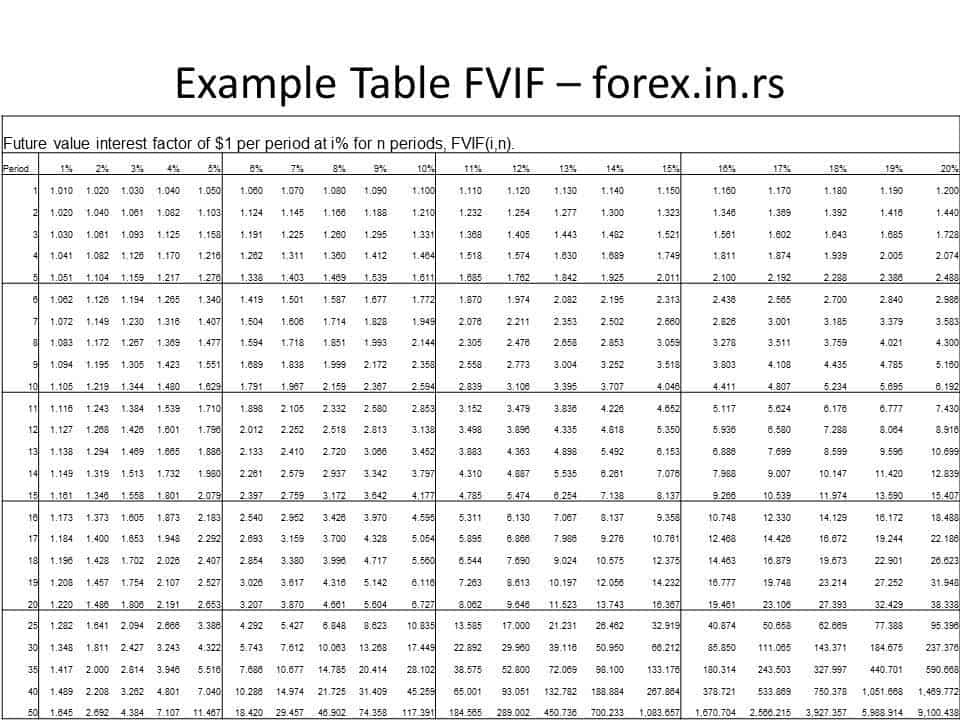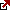# Future Value Factor

In finance, it is implied that the value of the money will increase over time if invested properly. The value of money received at present is more than the value of money that has to be received at a later date. This concept of money is based on the fact that an investor can invest the money he has received in the various options and earn some more money in the form of interest or dividends, or capital gain after some time. If he receives the money later, he will not earn the income he would have otherwise got till the receipt of the money after a year or more. Hence investors wish to calculate the value of money available with them at present at a future date.

Definition

What is the future value factor?

The future value factor (FVF) or future value interest factor (FVIF) is the equivalent value at some future date of a cash flow at time 0 or a series of cash flows that occur after an equal time interval. In simple words, the Future Value Factor or future value interest factor is used to calculate the value of a specific amount at a future date. Investors use this factor to estimate the value of the money they have at a future date, assuming a certain interest rate. It is also used for calculating the annuity payments or annuity due. For calculating the FVF, the interest is compounded based on the period specified. It is assumed that the principal’s interest during the period for compounding will also be reinvested and earn interest. Either a formula or tables can be used for determining FVF.

FVF Formula – How to calculate future value factor?

The formula for calculating the FFV uses the interest rate in percentage

FVF = ( 1 + r)^n

where r is the interest rate in percentage for the period after which it is compounded

n = number of periods for which the investment is compounded.

The investment’s annual interest rate should match the interest rate for each period and the number of periods when the interest is compounded.

an alternate formula for calculating the FVF uses the number of compounding periods in a year

FVF = (1+ (APR/m))^nXm

In this alternate formula, APR is the annual percentage rate for the investment, m is the number of periods in the year when the interest is compounded, and n is the total number of years. Similar formulas with some variations are used for calculating the FVF for the annuities, annuity due to FVF Table.

Since calculating the FVF using the formula is time-consuming and tedious as it involves compounding, many investors prefer to use tables to determine the FVF for different interest rates and periods. The table is usually available for 1 to 30 periods depending on the duration of the investment. Since the interest rate on debt is usually limited to a maximum of 18% only for most investments, the FVF corresponding to interest rates up to 18% are usually provided in the table. However, it is possible to generate tables with more periods and higher interest rates depending on the investment considered.

Future value interest factor Table example :Using the table for determining the FVF is comparatively simple; the investor will only have to look at the interest rate and the compounding period. The table is also convenient since the investor can easily compare the FVF for the different periods and different interest rates to make a decision accordingly; he does not have to calculate values manually. Similar tables are also available for annuities and annuity due. Most financial consultants will use the tables while advising the investor on the options available for investing their money.

Explanation

The FVF is used to determine the value of one dollar invested later for different interest rates and compounding periods. The factor obtained by calculation using the FVF formula or derived from the FVF table is then multiplied by the actual amount available. Investors can use this to choose between debt investments, which are less risky and offer low returns. Equity investments, which offer better returns yet may also result in losses for the investor. FVF highlights the time concept of money. It is better for a business or individual to get the payment due at the earliest to be invested, instead of waiting to receive the amount and losing the interest otherwise earned.

Latest posts by Fxigor (see all)

Fxigor

Igor has been a trader since 2007. Currently, Igor works for several prop trading companies. He is an expert in financial niche, long-term trading, and weekly technical levels. The primary field of Igor's research is the application of machine learning in algorithmic trading. Education: Computer Engineering and Ph.D. in machine learning. Igor regularly publishes trading-related videos on the Fxigor Youtube channel. To contact Igor write on: igor@forex.in.rs

Trade gold and silver. Visit the broker's page and start trading high liquidity spot metals - the most traded instruments in the world.